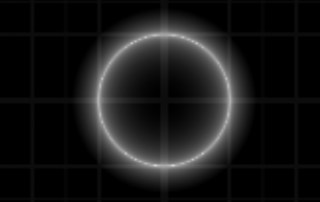## Category: equations and shapes

In the “equations and shapes” category, we post articles explaining the relations between equations and the shapes they define.

Sometimes, we will start with an equation, and ask for the corresponding shape; but we will also go the other way round: finding an equation for a certain shape.

We will encounter both the very basics – such as lines, circles, planes, and surfaces of balls (called spheres) – and more advanced and more interesting objects from the classical cubic surfaces to the modern Barth Sextic.

•Equations for lines and circles
Gallery

## Equations for lines and circles

This post explains briefly how lines and circles may be described by equations. A particular focus are implicit equations which will play an important role in later posts in the category "equations and shapes" on math-sculpture.com.

2018-12-03T23:50:24+01:00equations and shapes|
•Equations and Shapes
Gallery

## Equations and Shapes

This post explains briefly how lines and circles may be described by equations. A particular focus are implicit equations which will play an important role in later posts in the category "equations and shapes" on math-sculpture.com.

2018-12-03T23:52:03+01:00equations and shapes|